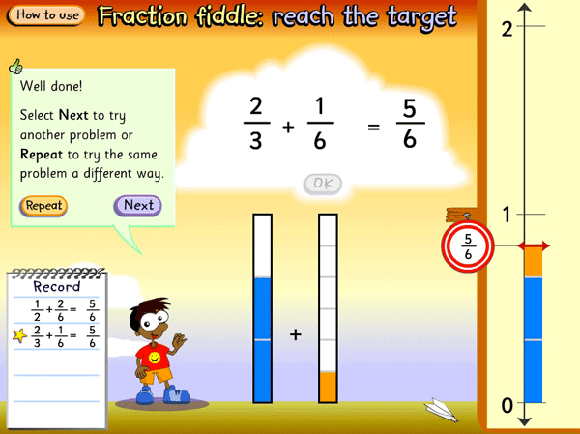# Reach the target

Demonstrate the learning object L2806 Fraction fiddle: reach the target to the class and discuss the dynamically linked components.

• A target number is presented (e.g. $$\frac{5}{6}$$) and two fractions that add to that target must be created.
• Make one denominator the same as the target denominator (e.g. sixths) and make the other denominator a factor or multiple of it (e.g. halves, thirds or twelfths).
• Adjust both numerators to create the desired fractions.
• As the fractions change, their fraction bars also change, as does the total on the vertical number line.
• Select <Repeat> and find another pair of fractions for the same total. Select <Next> for a new target number.Screen grab from L2806 Fraction fiddle: reach the target.
Source: © Education Services Australia Ltd, 2011

Pairs of students work together, taking turns to complete an addition task.

• Ask the students to print out the record sheet and compare solutions.
• Ask what strategies could be used to add fractions like these without the learning object to assist.

Students ready for a greater challenge can be encouraged to make both denominators different from the target fraction.